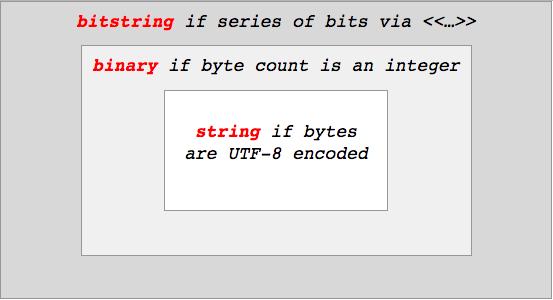# Bitstring and binary

Hi all

Could someone please explain me what is a bitstring?

And the difference between bitstring and binary.

Thanks

3 Likes

A bitstring is a type that stores arbitrary number of bits, you can have a 5bit bitstring whereas binary stores arbitrary number of bytes

Here is some code that should make things clearer:

``````# bitstring
bs = << 3 :: size(2) >>      # => 2 bits 11
IO.inspect bs                # => <<3::size(2)>>
IO.inspect is_bitstring(bs)  # => true
IO.inspect is_binary(bs)     # => false

# binary
bin = << 3 >>                # => 8 bits or 1 byte
IO.inspect bin               # => <<3>>
IO.inspect is_bitstring(bin) # => true
IO.inspect is_binary(bin)    # => true

``````

A binary is just a collection of bytes, so it has to have a number of bits that is divisible by 8 (i.e. a byte). So you can have a 8 bit binary, 16 bit binary and so on. If your binary is not divisible by 8, e.g. 7bits, 15bits, 14 bits, 23bits, you have a bitstring. And since a bitstring can have any number of bits even a binary is a bitstring. However, the inverse is not true.

5 Likes

If you want to have a deeper understanding of the subject and are willing to invest half an hour to appreciate it, I strongly recommend the following video:

ElixirConf 2016 - String Theory by Nathan Long & James Edward Gray II

Really useful, really interesting, loved every minute of it.

8 Likes

Thanks @jaysoifer! I also wrote some blog posts covering some of the talk topics in a little more depth:

@kostonstyle The way I phrased this distinction was:

In Elixir, a “bitstring” is anything between << and >> markers, and it contains a contiguous series of bits in memory. If there happen to be 8 of those bits, or 16, or any other number divisible by 8, we call that bitstring a “binary” - a series of bytes. And if those bytes are valid UTF-8, we call that binary a “string”.

So a subset of bitstrings are binaries, and a subset of binaries are strings. Like this:If you don’t understand what it means for something to be “UTF-8 encoded”, the first blog post should help.

17 Likes

Consider an example:

`a = << 3 >>`

Is a bitstring or binary, that contains number 3?

Since you do not specify a size for that given element it is assumed to be 1 byte. So the variable `a` does hold a bitstring of length 8 or a binary of length 1, and should be even a string (while not printable, it does only contain valid codepoints)

Remember the picture from above, every string is a binary and every binary is a bitstring, but not necessarily the other way round.

A bitstring is a binary if and only if it has a number of bits that is evenly devisiable by 8.
A binary is a string if and only if it does only contain valid unicode codepoints encoded in UTF-8.

So as you can see binary and string are true subsets of binary and string is a true subset of bitstring and binary.

3 Likes

It is both, a quick test shows

``````a = << 3 >>
IO.puts is_bitstring(a) # => true
IO.puts is_binary(a) # => true
``````

When you write `<< 3 >>`, there is an implicit `::size(8)` modifier added. So, `<< 3 >>` is the same as `<< 3 :: size(8) >>` and since the number of bits is divisible by 8, it is a binary and a bitstring

3 Likes